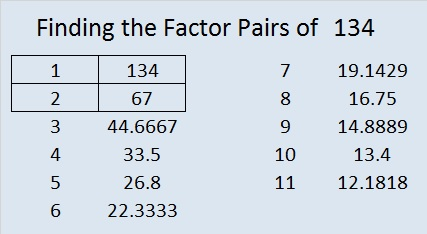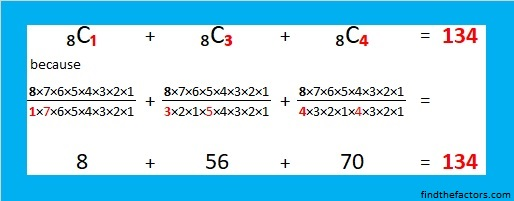# 134 and Level 1

134 is a composite number. 134 = 1 x 134 or 2 x 67. Factors of 134: 1, 2, 67, 134. Prime factorization: 134 = 2 x 67.Here is a Complicated but fascinating number fact from Wikipedia that involves 134 on BOTH sides of the equation:Notice that each term has an 8 sub-scripted on the left side of the C and either 1, 3, or 4 sub-scripted on the right side of the C. If you do all the division, multiplication and addition, you will discover that  CCC₄ = 8 + 56 + 70 = 134.

134 is never a clue in the FIND THE FACTORS puzzles.Excel file with puzzles and the previous week’s factor solutions: 12 Factors 2014-06-021.nerdinthebrain
2.ivasallay